# Tutorial: Create a Hypsometric Curve

 Site: Open Courseware for GIS Course: QGIS Advanced Tutorials Book: Tutorial: Create a Hypsometric Curve
 Printed by: Guest user Date: Sunday, 1 October 2023, 8:28 AM

## 1. Introduction

In this tutorial we're going to create a hypsometric curve. A hypsometric curve is a cumulative distribution function of elevations in a geographical area. It can be used to compare the geomorphology of catchments.

We're going to create a hypsometric curve for the Rur catchment using a tool from the Processing Toolbox and the DataPlotly plugin.

After this tutorial you're able to:

• Create a table with the cumulative distributions of elevations in a DEM
• Visualise the hypsometric curve with the DataPlotly plugin

## 2. Open project data from GeoPackage

1. Start QGIS

2. Open the project from the GeoPackage that is provided with this tutorial: from the main menu choose Project | Open From | GeoPackage...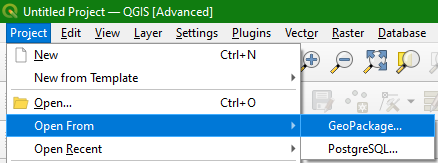3. In the Load project from GeoPackage dialogue browse to data_hypsometric_curve.gpkg as Connection and choose Hypsometric_Curve as Project and click OK.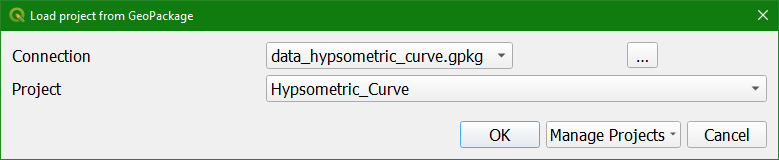Now you have a styled dataset consisting of a DEM raster layer and a catchment boundary polygon.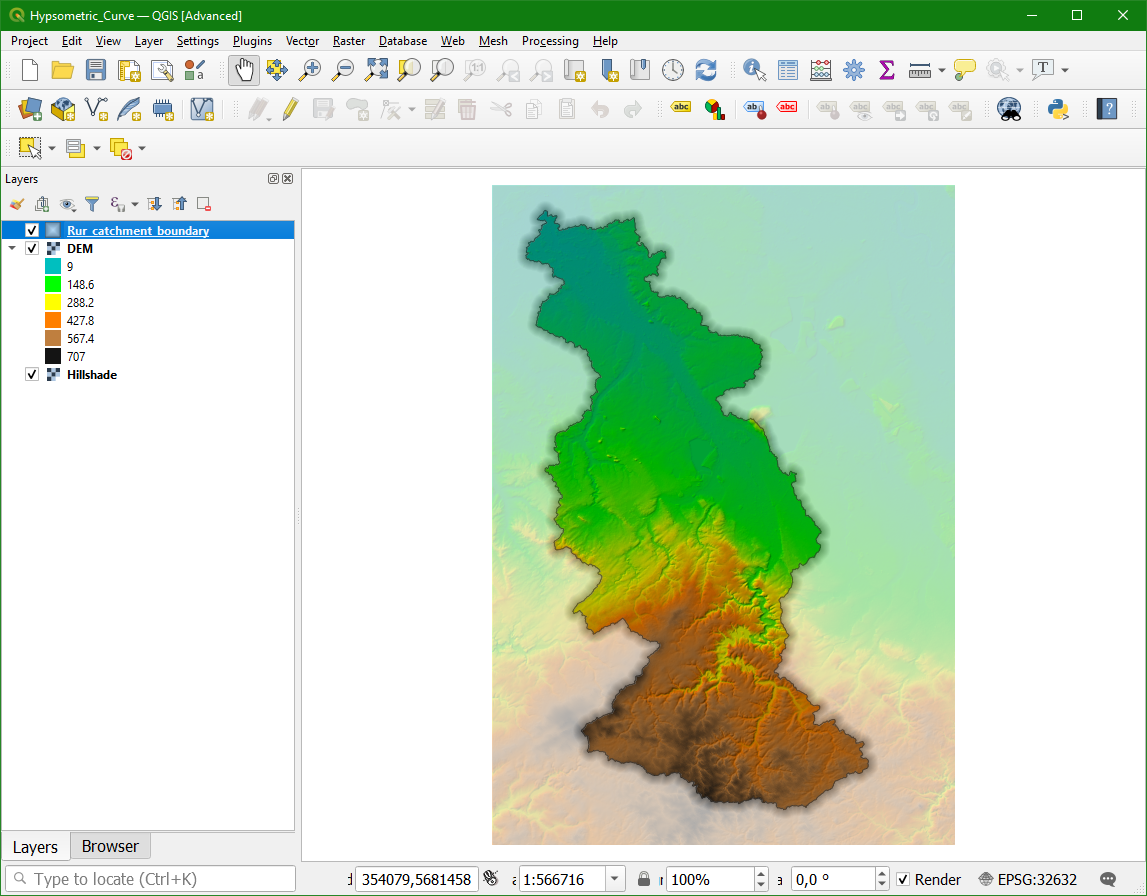In the next section we'll use that data to create a table with the cumulative elevation distribution in the catchment.

## 3. Create the cumulative elevation distribution table

In this section we're going to calculate the cumulative elevation distribution table.

1. Open the Processing Toolbox: from the main menu choose Processing | Toolbox.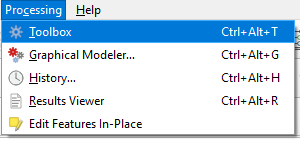2. In the Processing Toolbox choose Raster terrain analysis | Hypsometric curves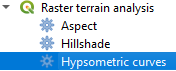3. In the Hypsometric Curves dialogue choose the DEM as DEM to analyze and Rur_catchment_boundary as the Boundary layer. The algorithm will only consider the elevation within this boundary layer. Change the Step to 50 meters. That is the elevation interval for which the cumulative percentages of the area will be calculated. To get the percentages check the box to Use % of area instead of absolute value. Choose an output folder to save the CSV (comma separated values) file and click Run.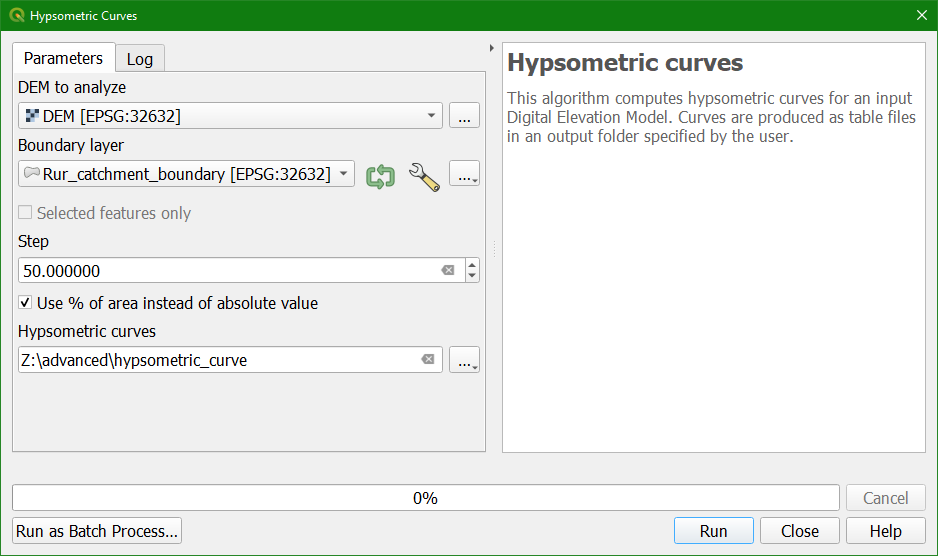4. Click Close when the algorithm has finished.

5. Go to the folder with and check if it has created histogram_Rur_catchment_boundary_1.csv. Inspect the file in a spreadsheet programme, such as LibreOffice Calc.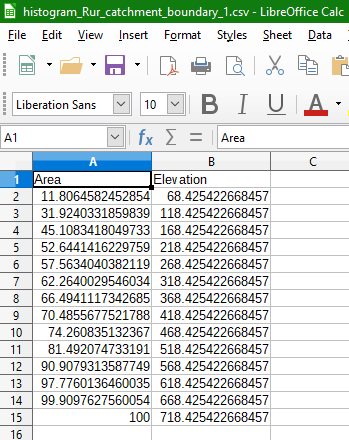There you can see in column A the areas in cumulative percentage of the total catchment area and in column B the elevations. So this means that 11.8% of the catchment has an elevation <= 68.5 m and 100% of the catchment has an elevation <= 718.4 m.

In the next section we'll create the curve from this table using the DataPlotly plugin.

## 4. Create the Hypsometric Curve with the DataPlotly Plugin

In this section we're going to create a curve from the data of the previous step.

1. Click the Open Data Source Manager icon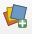and go to the Delimited Text tab.

2. Browse to the histogram_Rur_catchment_boundary_1.csv file. Under Geometry Definition choose No geometry (attribute only table). Click Add.